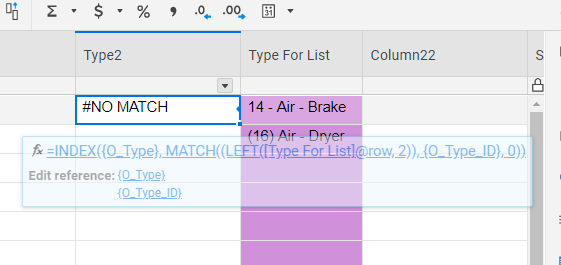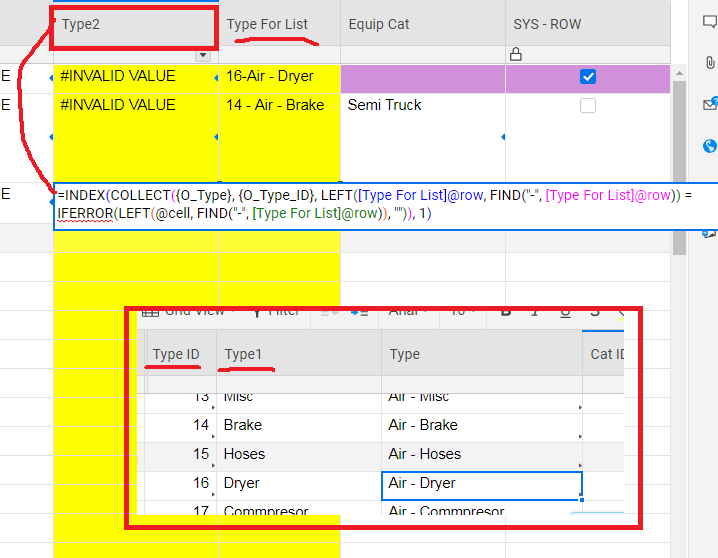# Index, Match with Find and Left function

I am trying to get a formula to look up a value with the INDEX / MATCH function and the Search_Value being the numbers up to a "-" dash from the "Type For List" column.

The index/match does not seem to like the LEFT or FIND functions

Has anyone come across this or has a work around?

=INDEX({O_Type}, MATCH((LEFT([Type For List]@row, 2)), {O_Type_ID}, 0))Tags:

• =index(collect({O_Type},{O_Type_ID},left(@cell,2) = LEFT([Type For List]@row, 2)),1)

* Untested

• =index(collect({O_Type},{O_Type_ID},find(LEFT([Type For List]@row,2),@cell)&gt;0,1)

Would be another option if you have different formats and just want to find the number

• reread the problem haha this one will search out the "-" save what is to the left of it, and search for it in your results column, and return the associated value

=index(collect({O_Type},{O_Type_ID},left([Type For List]@row,find("-",[Type For List]@row))=left(@cell,find("-",[Type For List]@row)),1)

*Untested

• thank you [email protected]

I tried the formula but can not get it to work.... #UNPARSEABLE

I have not used collect or @cell yet.

• Not really sure what is missing. Try this

=index(collect({O_Type},{O_Type_ID},left([Type For List]@row,find("-",[Type For List]@row))=iferror(left(@cell,find("-",[Type For List]@row)),""),1)

also, check your other sheet references. They need to be the same number of cells, which could cause the issue.

https://help.smartsheet.com/function/collect

https://help.smartsheet.com/articles/2476491-create-efficient-formulas-with-at-cell

• Found it. Missing a parenthesis

=index(collect({O_Type},{O_Type_ID},left([Type For List]@row,find("-",[Type For List]@row))=iferror(left(@cell,find("-",[Type For List]@row)),"")),1)

Tested Version that works (R = Return Column, S = Search Column, T = Value to parse for the search)

=INDEX(COLLECT(R:R, S:S, LEFT([email protected], FIND("-", [email protected])) = IFERROR(LEFT(@cell, FIND("-", [email protected])), "")), 1)

• Thank you very much for taking the time to help me with this formula.

I just can not get it to work for me... can you see what i am missing. I copied your tested formula and inserted my references/cell.• Alright thanks. That screenshot makes this a lot clearer. I misunderstood the layout of the sheets

=INDEX(COLLECT({O_Type}, {O_Type_ID}, @cell = value(LEFT([Type For List]@row, FIND("-", [Type For List]@row)-1))) , 1)

Test that out and let me know if it works for you.

• Thank you so much. Yes that formula works very nicelyVery much appreciated.

• Finally haha Took me long enough.

Not a problem